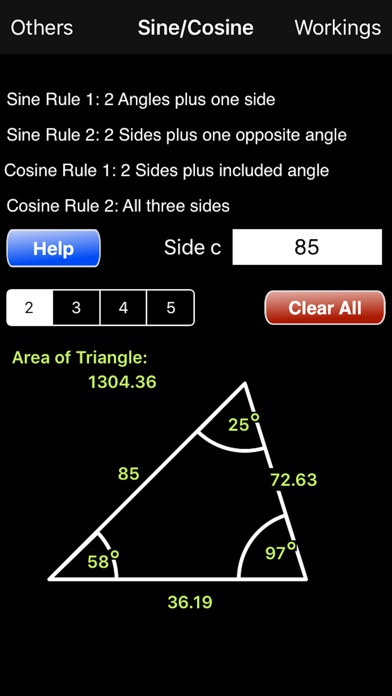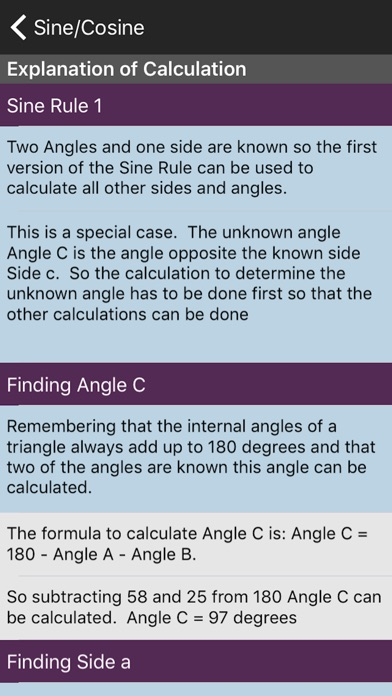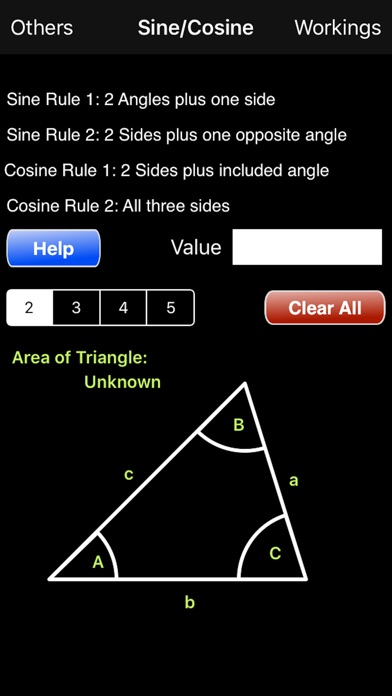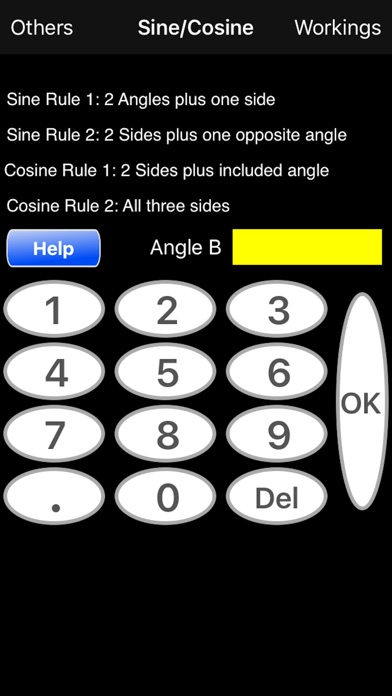SineCosine

iOS UniversalEducation

\$0.99

Do you need to learn how to determine the angles and lengths of a non-right-angled triangle? Need to understand how to apply the Sine and Cosine rules in this case? Need to know how to calculate the other sides and angles when you know one angle and two sides or two angles and one side or even no angles and all three sides? If the answer is Yes to any of these questions then this App is for you. The Sine/Cosine Calculator not only calculates the answers for you but it also tells you how it did it so you can understand how and when to apply the rules for non-right angle triangles.

The App is simple to use. Just enter the known angles and sides and all the others will be calculated for you. To see how the answers were arrived at just select the 'Workings' button and a page is shown with a comprehensive set of steps to show how each was calculated.

To enter a value for an angle or a side just touch the diagram on or near the one you want to specify. The keypad pops up. Enter the value you want and select OK.

The App can calculate the answers if you specify a) two angles and a side, b) two sides and the opposite angle, c) 2 sides and the included angle or d) all three sides.

To start over select 'Clear All'. All values are reset.

••••